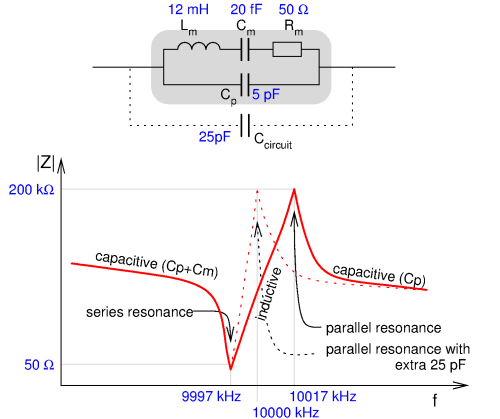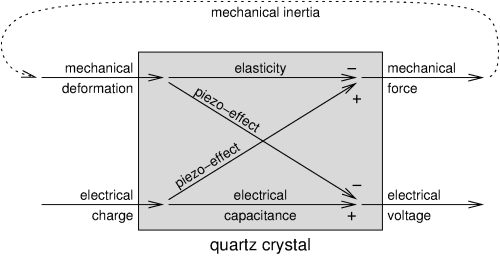# Quartz crystals

Pieter-Tjerk de Boer, PA3FWM pa3fwm@amsat.org

(This is an adapted version of part of an article I wrote for the Dutch amateur radio magazine Electron, August 2016.)Quartz crystals have been used in radio technology since the 1920s, to build very stable oscillators and to filter signals. In WW2 they started to be mass-produced, as is very nicely documented in , back then based on natural quartz from Brazil. Nowadays quartz crystals are still a mass product (more than 2 billion per year, according to Wikipedia), most of which spend their lives humbly clocking a microprocessor. But the importance of even that task should not be underestimated: according to  a train crashed in 1972 due to a badly designed crystal oscillator spontaneously jumping to its third overtone.

Such a crystal is nothing more than a slice of quartz, sawn under the right angle from a (nowadays synthetic) crystal, with an electrode on both sides. The picture shows two common crystals with frequencies of a few MHz; usually such crystals are in metal enclosures, but sometimes one encounters these glass versions. These crystals use the so-called thickness-shear vibration, in which both surfaces of the crystal plate shift w.r.t. each other, as sketched at the right. The two crystals above are much larger and resonate at lower frequencies, alternately getting longer and shorter. There are also crystals which resonate by bending like a tuning fork; among others the small 32.768 kHz crystals in watches work like this.The next figure shows the well-known equivalent circuit of a crystal, with in blue typical values for a 10 MHz crystal. The inductance Lm and the capacitance Cm are called the "motional inductance" and "motional capacitance", because they are directly related to the motion of the crystal (as will be discussed further down the page). Cp is the parallel capacitance: the electrodes on the crystal form a small capacitor. And Rm is a resistor which represents the losses (due to friction etc.). The frequency on which the series circuit of Lm and Cm resonates is called the series resonance: at this frequency the crystal has a very low impedance, practically only Rm (with Cp in parallel, but that has little effect). In contrast, the impedance becomes very high at the parallel resonance frequency: there Lm resonates with the series circuit of Cm and Cp connected in parallel to it. Their total capacitance is slightly less than Cm alone, so the parallel resonance frequency is a little higher than the series resonance. In practice, parallel to Cp there is the capacitance of the circuit to which the crystal is connected, which does not have an influence on the series resonance, but does affect the parallel resonance. That is why crystals for parallel resonance are always specified for a specific extra parallel capacitance; in this example, 25 pF is needed to bring the crystal to 10.000 MHz, as shown in dotted lines.

## How does a quartz crystal resonate?

At first, the answer to this question is simple: quartz is slightly elastic, so it can vibrate in the same way e.g. a rubber band or guitar string can vibrate. You start with pulling the rubber band from its neutral position. Due to this deformation, a force arises in the elastic material which tries to undo the deformation. Next, you let go of the rubber band. The material starts to move towards its neutral position, the force decreases and becomes zero when the neutral position is reached. By then however, the material is in motion at some speed and will "overshoot" to the other side of the neutral position. The elastic force will work against this, and so on. We typically don't imagine a crystal as elastic, but the principle is the same. Only the force becomes very large already at a very small deformation, so the vibration will be much faster (higher frequency) than with a rubber band.However, in a quartz crystal there are not just mechanical effects as discussed above; due to the piezo-electric effect, also electric effects play a role. On both sides of the crystal there's a metal layer, between which a voltage can exist, and/or on which a charge can accumulate. The relationship is illustrated in the figure. We see the crystal as a "black box", with two kinds of "input" at the left and two kinds of "output" at the right. The inputs are how much the crystal is deformed, and how much electrical charge has been put onto the connection plates. The outputs are the force in the crystal which acts against the deformation, and the electrical voltage we measure across the crystal. How these outputs depend on the inputs is determined by physical properties of the crystal, and is indicated in the figure.

B.t.w., this model is not the only possibility. One can also choose different combinations of input and output, e.g., take the force as input and the resulting deformation as the output, and there is also some freedom in the choice of plus and minus signs (i.e., which direction is denoted positive). But the model as shown here, is the handiest for explaining the resonance.

Let's first assume that there is no charge on the crystal. The crystal is not connected to anything, but we do deform it. We see that that deformation causes two effects: a mechanical force and and an electric voltage. That mechanical force gets a minus sign, indicating that it works against the deformation.

Let's next assume that we have not deformed the crystal, but using an electrical current we have put some charge on the electrodes. We see that also in this case there will be an electical voltage and a mechanical force. The electrical voltage should not surprise us: the whole thing looks like a capacitor, and when charge is fed to a capacitor, a voltage develops across it. The mechanical force is the piezo-electric effect, and causes the crystal to "want" to deform itself.

What happens if we apply alternating current? By the above argumentation, there will be an alternating mechanical force in the crystal. If the frequency is very high, that force is not capable of significantly moving the crystal, due to its inertia: the deformation remains nearly zero. Electrically the whole thing then still works as a capacitor: Cp in the equivalent circuit.

If the alternating current has a very low frequency, the crystal can start moving due to the piezo-effect's mechanical force: the crystal will be deformed. That deformation in turn will again cause an electric voltage and a mechanical force. Due to the plus/minus signs in the figure this effect is such that both the electrical voltage and the mechanical force will be smaller in total than when there was no deformation. Thus, we see that at low frequencies the same amount of charge results in a lower voltage than at higher frequencies: the thing still behaves as a capacitor, but with a (slightly) higher capacitance. That matches the equivalent circuit: for low frequencies the inductor does nothing, so Cm and Cp are in parallel.

Next, consider the crystal with nothing connected to it. We deform it a little bit and then let go. The mechanical force tries to undo the deformation, but due to inertia the deformation "overshoots" in the opposite direction, which again will be counteracted by the elastic force, and so on: the crystal vibrates mechanically on its own resonant frequency. The deformation alternates between positive and negative values, and according to the figure this also leads to an alternating voltage across the crystal. All of this happens without us applying any charge, i.e., without any electrical current. So there is an AC voltage, but no current: on this frequency apparently the crystal has a high impedance: it's the parallel resonance.

Finally, consider the situation in which we short-circuit the crystal electrically, again apply an initial deformation, and let go. The short circuit (direct wire between the crystal's metal plates) ensures that no voltage can develop across the crystal. As soon as a voltage "tries" to arise due to deformation and the piezo-effect, a current will flow and transport charge from one plate to the other. That charge in turn also causes a voltage, and the system will constantly search for an equilibrium in which just so much charge is displaced that the total voltage is 0 (because no voltage can exist, due to the short-circuit connection). But that displaced charge in turn also causes a contribution to the mechanical force, and according to the plus/minus signs in the figure that force will be opposite to the elastic force that is caused by the deformation itself. Thus, the net effect of short-circuiting the crystal is that the crystal is pushed slightly "less strongly" back to its neutral state, and thus that the crystal resonates at a lower frequency than without the short circuit. At this frequency there is no voltage across the crystal, while there is a non-zero current: that's a low impedance. This is the series resonance.

Thus, we see how the mechanical resonance of the crystal can be recognized in how its impedance depends on the frequency, and how the mechanical resonance moves to a slightly different frequency when we short-circuit the crystal.

## References:

 Youtube movie: Crystals go to war
 https://web.archive.org/web/20090312102803/http://www.edn.com/article/CA6636507.html

Text and pictures on this page are copyright 2016, P.T. de Boer, pa3fwm@amsat.org .
Republication is only allowed with my explicit permission.